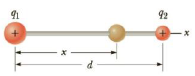Chapter 15, Problem 66AP

Chapter
Section
Textbook Problem

Two small beads haring positive charges q1 = 3q and q2 = q are fixed at the opposite ends of a horizontal insulating rod of length d = 1.50 m. The bead with charge q1 is at the origin. As shown in Figure P15.66, a third small charged bead is free to slide on the rod. At what position x is the third bead in equilibrium?Figure P15.66

To determine
The position x.

Explanation

Given info: The charges on the beads are q1=3q and q2=q . The length of the insulating rod is d=1.50m . The third bead is kept at distance x from q1 .

The free body diagram is,

• F1 is the electric force due to the charge q1=3q .
• F2 is the electric force due to the charge q2=q .

Formula to calculate the electric force due to the charge q1=3q . is,

F1=ke(3q)(Q)x2

• ke is the Coulomb constant.
• Q is the charge on the third bead.

Formula to calculate the electric force due to the charge q2=q

Still sussing out bartleby?

Check out a sample textbook solution.

See a sample solution

The Solution to Your Study Problems

Bartleby provides explanations to thousands of textbook problems written by our experts, many with advanced degrees!

Get Started